# AP Chemistry : Reduction Potential

## Example Questions

### Example Question #1 : Reduction Potential

The standard reduction potentials for certain metals are listed below: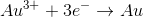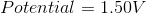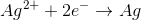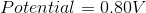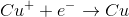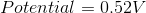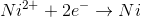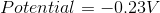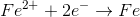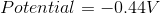What is the standard potential of a cell in which copper is reduced by iron?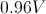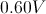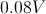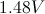Explanation:

In order to find the potential of a galvanic cell in which copper is reduced by iron, we need to combine the two half reactions for the metals in question.

Since copper is reduced in the net ionic equation, we can use the reduction potential seen in the table.Iron must be oxidized in order to reduce the copper. As a result, we must use the reverse reaction in the table. Remember that the table only gives reduction potentials; you will frequently need to charge the reaction to find an oxidation potential.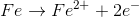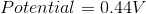Notice how the potential for the half reaction has been switched as well. Combining these two reactions gives the following balanced reaction, once the electron transfer is balanced.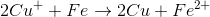Reduction potentials are intensive properties, so we do not multiply the potential of the copper half reaction by two. The total potential is simply the addition of both half reaction potentials.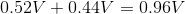### Example Question #2 : Reduction Potential

The standard reduction potentials for some metals are listed below: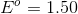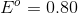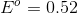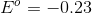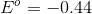Which of the following metals is the strongest reducing agent?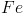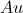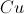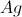Explanation:

The reducing agent is the metal that will be oxidized in the reaction. Since all of the half reactions shown are reduction potentials, we need to switch the half reactions in order to determine which results in the greatest cell potential when the metal is oxidized. Iron will result in a cell potential of 0.44 volts when oxidized, which makes it the strongest reducing agent on the list.

### Example Question #3 : Reduction Potential

Standard Reduction Potentials

Cr3+(aq) + 3e → Cr (s)          –0.74 V

Cu2+(aq) +2e  → Cu (s)       0.34 V

Consider the following reaction

Cu(s) + Cr3+(aq) ⇌ Cu2+(aq) + Cr(s)

What is the Eo Cell for the reaction?

–1.08 V

1.08 V

0.46 V

–0.40 V

–1.76 V